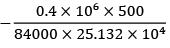Courses

# Test: Torsion Level - 3

## 10 Questions MCQ Test Strength of Materials (SOM) | Test: Torsion Level - 3

Description
This mock test of Test: Torsion Level - 3 for Mechanical Engineering helps you for every Mechanical Engineering entrance exam. This contains 10 Multiple Choice Questions for Mechanical Engineering Test: Torsion Level - 3 (mcq) to study with solutions a complete question bank. The solved questions answers in this Test: Torsion Level - 3 quiz give you a good mix of easy questions and tough questions. Mechanical Engineering students definitely take this Test: Torsion Level - 3 exercise for a better result in the exam. You can find other Test: Torsion Level - 3 extra questions, long questions & short questions for Mechanical Engineering on EduRev as well by searching above.
QUESTION: 1

### Two shafts are made of the same material and same outer diameter. With one having a circular section, and the other a hollow circular section with inner diameter, half that of outer diameter. The torque that can be transmitted by the solid section is N times that of the hollow section, where N is

Solution: Given d = D /2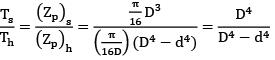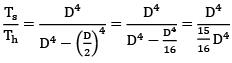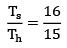Ts =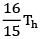N = 16/15

QUESTION: 2

### A solid phosphor bronze shaft 60 mm in diameter is rotating at 800 rpm and transmitting power. It is subjected to torsion only. An electrical resistance strain gauge mounted on the surface of the shaft, gives the strain reading as 3.98 × 10−4 . If the modulus of elasticity for bronze is 105 GN/m2 and Poisson’s ratio 0.3. The power transmitted by the shaft will be (Bending effect may be neglected) (Gage is mounted at 45o to the shaft axis).

Solution: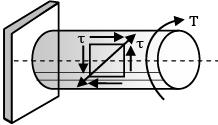p1 = +τ, p1 = −τ strain gages

Internal at + 45° to axis.

Strain, ϵ =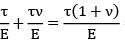= 3.98 × 10−4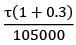= 3.98 × 10−4

τ, maximum shear stress =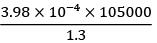=32.146 N/mm2

Torque,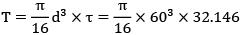= 1363366 Nmm = 1363.36 Nm

rpm, N = 800 Angular speed,

ω =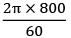Power = ωT = 83.78 × 1363.36 Nm/s = 114216.6 W

= 114.216 kW

QUESTION: 3

### A round shaft of diameter 'd' and length 'l' fixed at both ends 'A' and 'B' is subjected to a twisting moment ‘T’ at 'C', at a distance of l/4 from A (see figure). The torsional stresses in the parts AC and CB will be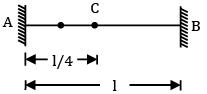Solution: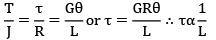τACLAC = τCBLCB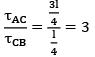QUESTION: 4

A horizontal shaft, L m long, is rigidly fixed at both the ends. At section C and D, torques 3 kNm (ccw) and 5 kNm (ccw) looking from the side A are applied. What are the fixing couples TA and TB?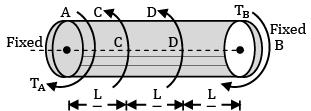Solution: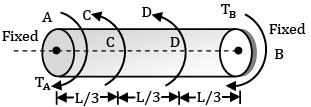There are 3 portions AC, CD and DB, each of length L/3

Torque at A, say TA

Torque between CD = TA − 3kNm

Torque between DB = TA − 3 − 5

= TA − 8 kNm

Angular twist θAB = 0, since both the ends are fixed

θAC + θCD + θDB = 0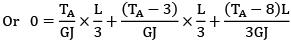or TA + TA − 3 + TA − 8 = 0

3TA = 11

TA = 11/3 = 3.667 kNm

TA − 3 = 0.667 kNm

TA − 8 = −4.333 kNm = TB

Torque distribution is shown in figure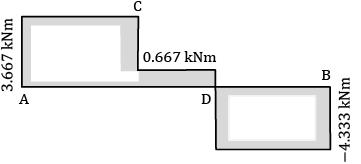QUESTION: 5

In a torsion test, the specimen is a hollow shaft with 50 mm external diameter and 30 mm internal diameter. An applied torque of 1.6 kNm is found to produce an angular twist of 0.40° measured on a length of 0.2 m of the shaft. Young’s modulus of elasticity obtained from the elastic test has been found to be 200 GPa.

Then the Poisson’s ratio will be

Solution: Strain T = 1.6 kNm = 1.6 × 106 Nmm θ = 0.40 =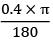= 6.98 × 10−3 rad

L = 200 mm d = 50 mm Polar moment,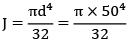= 61.38 × 104mm4

Shear modulus,

G =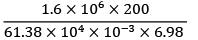= 74.7 × 103 N/mm2 (modulus of rigidity)

= 74.7 GPa

E = 2G[1 + ν] ν, Poisson’s ratio

=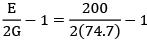= 0.338

QUESTION: 6

Two beams are of same length and same weight. One beam is of solid circular section and the other is a hollow section. The internal diameter is half of the external diameter. The ratio of the strength of hollow section to that of solid section is

Solution: Solid and hollow sections have same weight Wh = Ws γ × Vh = γ × Vs

Ah = As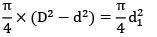D2 − d2 = d12

Assume k = d / D

D2(1 − k2 ) = d12

D √(1 − k2) = d1 ⋯ ①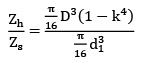=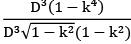=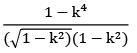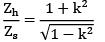=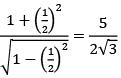=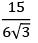QUESTION: 7

A circular section rod ABC is fixed at ends A and C. It is subjected to torque T at B. !B = BC = L and the polar moment of inertia of portions AB and BC are 2 J and J respectively. If G is the modulus of rigidity, what is the angle of twist at point B?

Solution: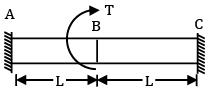θAB = θBC

or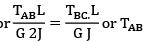= 2TBC

TAB + TBC = T or TBC = T/3

or QB = QAB =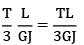QUESTION: 8

A solid circular rod AB of diameter D and length L is fixed at both ends. A torque T is applied at a section x such that !X = L/4 and BX = 3L/4. What is the maximum shear stress developed in the rod?

Solution: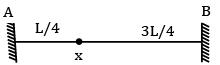θAX = θXB and TA + TB = T

or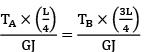or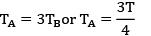τmax =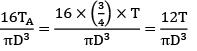QUESTION: 9

A hollow shaft having an inside diameter 60% of its outside diameter is to replace a solid shaft transmitting the same power at the same speed. If the material is the same, the percentage saving in the materials is

Solution: Torque transmitted by solid shaft

T =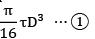Torque transmitted by hollow shaft

T =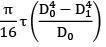=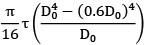=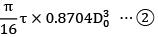Torque transmitted is the same equate ① and ②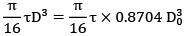D = 0.9548 D0

Area of solid shaft. As =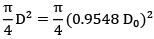= 0.716 D02

Area of hollow shaft,

AH =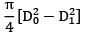=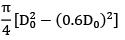= 0.502 D02

For the same material, the weight of the shaft is proportional to the areas Saving in material = saving in area

=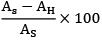=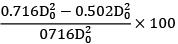= 29.88%

QUESTION: 10

A stepped shaft ABC, 1m long made of steel is of diameter 20 mm from A to B = 0.5m, 40 mm from B to C = 0.5 m. It is subjected to a twisting moment of 0.4 kNm (cw) at A, 0.8 kNm (ccw) at B and 0.4 kNm (cw) at C. If G = 84 N/mm2, the angular twist between A and C. (in degrees) is ______________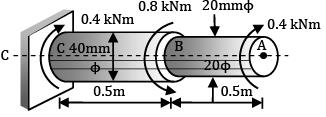Solution:

!B Torque = 0.4 kNm = 0.4 × 106 Nmm L = 500 mm

J =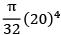= 1.5708 × 104 mm4

θAB =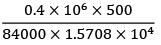BC Torque = −0.4 kNm = −0.4 × 106 Nmm L = 500 mm

J =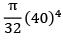= 25.132 × 104 mm4

θBC =